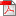# The effects of $O(\alpha^2)$ initial state QCD corrections to $e^+e^- \rightarrow \gamma^*/Z^*$ at very high luminosity colliders

Johannes Bluemlein (DESY)
DESY Auditorium, 16:45 h

We present the recently completed O(α2) initial state corrections to the process e+e− → γ*/Z*,
which is a central process at past and future high energy and high luminosity colliders for
precision measurements of the properties of the Z-boson, the Higgs boson, and the top quark.
We observe differences to an earlier result in the non-logarithmic contributions at O(α2).
The new result leads to a 4 MeV shift in the Z width considering the lower end s0 = 4 m2τ of
the radiation region, which is larger than the present accuracy. We present predictions on the
radiative corrections to the central processes e+e- → γ*/Z*, e+e- → Z H and e+e- → t t planned
at future colliders like the ILC, CLIC, Fcc-ee and CEPC to measure the mass and the width of the
Z boson, the Higgs boson and the top quark, for which the present corrections are significant.Poster (438KB)
Poster